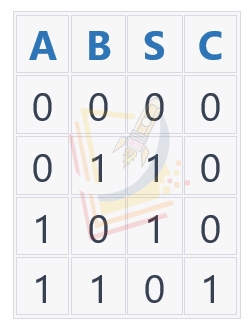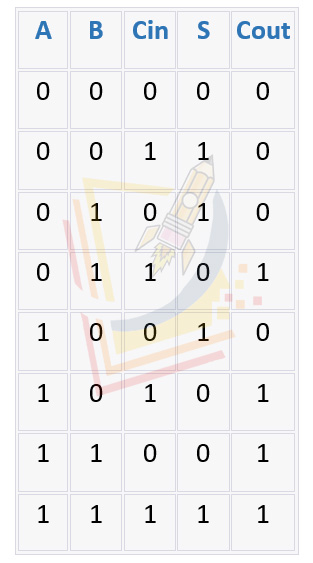# INTRODUCTION

Combinatorial logic circuits are digital circuits that perform logical operations on a set of inputs to produce a set of outputs. These circuits are called combinatorial because their outputs depend only on the current state of the inputs, and not on any previous inputs or outputs. Two of the most basic combinatorial logic circuits are the half adder and the full adder.

A half adder is a combinatorial circuit that performs the operation of addition of two single binary digits (bits). It has two inputs, A and B, and two outputs, S (sum) and C (carry). The truth table for a half adder is as follows:As we can see from the truth table, the output S is the XOR of inputs A and B. The output C is the AND of inputs A and B.